# Magnetized Material Notes | Study Electromagnetic Fields Theory - Electronics and Communication Engineering (ECE)

## Electronics and Communication Engineering (ECE): Magnetized Material Notes | Study Electromagnetic Fields Theory - Electronics and Communication Engineering (ECE)

The document Magnetized Material Notes | Study Electromagnetic Fields Theory - Electronics and Communication Engineering (ECE) is a part of the Electronics and Communication Engineering (ECE) Course Electromagnetic Fields Theory.
All you need of Electronics and Communication Engineering (ECE) at this link: Electronics and Communication Engineering (ECE)

Magnetized Material

In dealing with electrostatics, we had seen that the electrical polarization of the medium itself influences the electrical behavior of substances. In the same way we have internal currents within a material because there are moving charges in atoms and molecules which can create their own magnetic field.

There are two types effects here, the first is due to the orbital motion of the electrons and the second is due to the intrinsic spin magnetic moment of the electrons. The second effect is purely quantum mechanical in origin and its discussion is beyond the scope of this course. Like in the electrostatic case, the magnetic moment could be intrinsic to an atom or molecule or it could be induced by an externally applied magnetic field.

We will see later that in terms of theor magnetic behavior, substances fall broadly into three classes, viz. diamagnets, paramagnets and ferromagnets. Diamagnetism arises because of electromagnetic induction effect where the atomic currents oppose any change of flux through the atoms by an external magnetic field. We will discuss this effect later in more detail. The total current inside a material consists of two parts, the first is the conduction current, which is due to bodily motion of the free charges in a conductor and the second is due to atomic currents. The latter are too tiny to be taken care of individually and we consider such effects in an average sense.

Let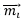be the magnetic moment of the i-th atom inside a matter. We define magnetization as the net magnetic moment per unit volume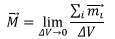The quantity is very similar to the polarization in dielectric material. We will calculate the effect due to the magnetization of the material by calculating the vector potential corresponding to the microscopic currents. We had seen earlier that the magnetic vector potential due to a magnetic moment is given by the expression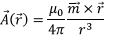where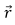is the position vector of the point of observation with respect to the position of the magnetic moment.

Using this we can write down the expression for the vector potential at a positiondue to magnetic moments I n a magnetized material having a magnetization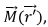where, as before,

we have used the primed quantities to indicate the variable to be integrated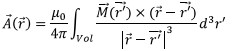As we did in the electrostatic case, we can convert this into two integrals, one over the volume and the other over the surface of the material, We can rewrite the vector potential as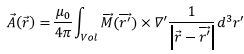We now use the vector identity for the curl of a product of a scalar with a vector,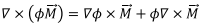using which we can write,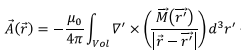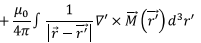The first term can be converted to a surface integral in a manner very similar to the way we converted volume integral of a divergence to a surface integral,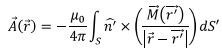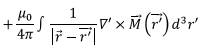Thus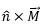takes the role of a surface current. We now identify, as we did in the electrostatic case, a bound volume current and a bound surface current, defined by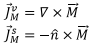The origins of these currents can be understood by realizing that there are large number of internal currents within the material which may not cancel within the volume. The surface term is understood similar to the way the polarization charge was introduced as even when internal currents cancel out, the currents at the boundary do not have neighbouring loops to cancel. Let us calculate the magnetic field due to magnetization of the material.

Let us start with the expression for the vector potential. If the gradient is taken with respect to the point of observation, we can write,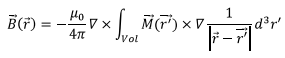We use the identity,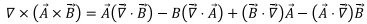where A and B are arbitrary vector fields not to be confused with the vector potential and the field respectively.

Using this identity, we have,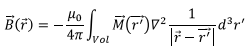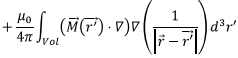Since the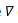operators are with respect to the unprimed index,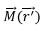is not differentiated with respect to it.

Using the fact that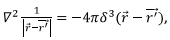we can perform the first integration and are left with,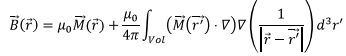The last term is expressed in terms of gradient of a vector which is to be understood, as explained earlier as gradient of three components of the vector on which it acts. Using the vector identity stated above, and, using the fact that the operatordoes not act on vectors or scalars which are functions of primed indices, we can write the second term of the above equation as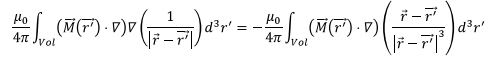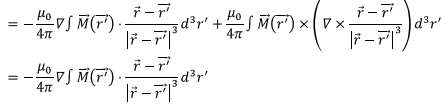the last line follows because the curl in the previous expression is zero. Thus we get, for the magnetic field,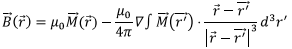Since curl of a gradient is zero, we get,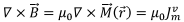If we add the conduction current contribution to the above, we get Ampere’s law for the combined case to be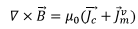The integral form of the Ampere’s law follows,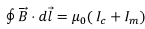where the magnetization current is defined in terms of the magnetization current density,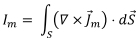We can rewrite Ampere’s law as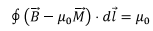which now includes the contribution due to the magnetic medium. We now define, a new vector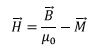which, incidentally, is also known as the magnetic field. There is a lot of confusion in literature because of this. The B field that we have been using so far, has been called the “magnetic field of induction” or the “magnetic flux density” in literature. However, whenever there is no confusion we will call each of these fields as the magnetic field and the context should make it clear which field we are referring to. When both are used in the same discussion, we will simply call them as the B field or the H field. The line integral of the H- field gives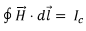The situation is very similar to the use of E and D in electrostatics. The H field tries to artificially separate the contribution due to the applied magnetic field and is thought to arise from the conduction current alone. This is artificial because there is no physical way in which one can achieve this separation and what an experiment would measure is the B field which includes both the contributions. Note that there is a dimensional difference between H and B, the former is measured in units of A/m.

Using Stoke’s theorem, we can write the curl of H as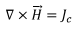This enables us to relate H to the scalar magnetic potential by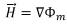If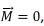we can write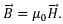For small applied field the system has a linear response to the field, i.e.,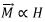so that we can write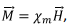, the proportionality factor Xis known as the magnetic susceptibility of the material. As we stated earlier, there are three basic type of magnetic material. There are substances which are known as “paramagnetic” for which the magnetic susceptibility is positive while for “diamagnetic” material , it is negative. There is a third class of material known as “ferromagnets” which show hysteresis behavior. For substances which show linear response, we can write,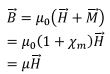where 1+Xis known as “relative permeability” .

Boundary conditions on B and H fields are obtained by using tricks similar to that used earlier. Gauss’s law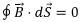leads to the normal component of magnetic field of induction being continuous at an interface and the Ampere’s law for the H field,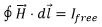leads to a discontinuity in the tangential component of the H field at a surface having a surface current,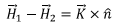Example : A Uniformly Magnetized Sphere

We will use the scalar potential concept to discuss this case. Recall that the scalar magnetic potential for a magnetic moment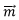is given by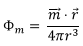This is very similar to the corresponding electrostatic expression for the scalar potential due to an electric dipole moment,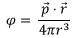Thus, following the way we obtained an expression for the scalar potential for a dielectric material and separated the contribution into two parts, a volume part and a surface part, we can write,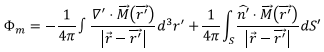(This is like defining a “magnetic charge density” with a volume component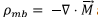and a surface component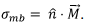In reality, magnetic charges do not exist but it helps drawing parallel to the electric case.)

In this case, since the magnetization is uniform, the volume component vanishes and we are left with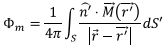The normal to the surface being in the radial direction, if we take the direction of magnetization along the z direction,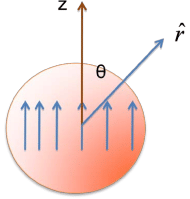we have,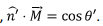We will expand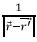in spherical harmonics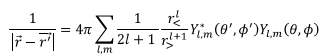Where the primes quantities refer to coordinates of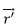and the unprimed quantities to coordinates of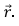Note that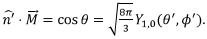The surface integral is essentially an integration over the solid angle which picks up, because of orthogonality condition on spherical harmonics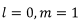term only, leaving us with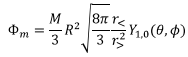Inside the material,  r > = R, r<= r  which gives for the magnetic potential inside,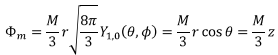Outside the sphere, r>= r, r<=rR and we get,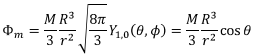Using these, we can get expressions for the magnetic field both inside and outside. Inside, since the scalar potential only depends on z,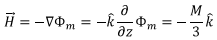which gives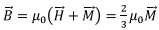Outside the sphere, we use spherical polar coordinates and since there is no azimuthal dependence of the potential, we have,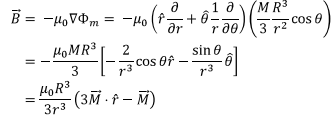Which is a familiar expression for the magnetic field outside.

The field inside is uniform while the field outside must form closed loops.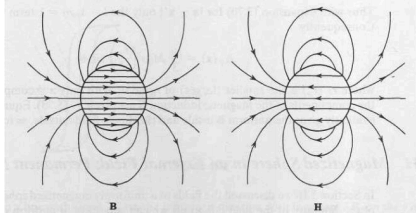Tutorial Assignment

1. A long cylindrical rod of radius R has a uniform magnetization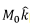parallel to its axis. There are no free currents. Find the magnetic field both inside and outside the cylinder due to magnetization.
2. A cylindrical wire, made of a linear magnetic material of permeability μ has a current | distributed uniformly over its cross section of radius R. Calculate all the bound current densities and determine the total current

Solutions to Tutorial Assignments

1. Since the magnetization is uniform, there are no bound volume currents. There will however be bound surface currents, given by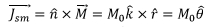Since the current is in the (azimuthal) direction of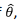the problem is similar to that of a solenoid. The field outside the cylinder is zero but the field inside is along the axis and is given by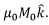2. As the free current is given, we can use Ampere’s law to determine the H field, which is given by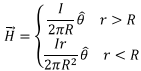Since the material is linear we can write the corresponding B field by multiplying the field inside by μ and the outside field by μ0 i.e.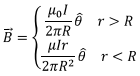Thus the magnetization vector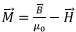is non zero only inside the material and is given by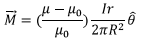The volume bound current density is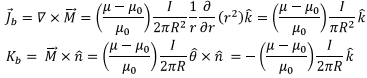Since the magnitudes of the bound current densities are constant, the total bound current from volume is obtained by multiplying the current density with πR2 and the surface bound current is obtained by multiplying with the circumference 2πR. These two contributions being equal and opposite, the net bound current is zero.

Self Assessment Questions

1. A long cylindrical rod of radius R has a magnetization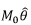in the azimuthal direction. There are no free currents. Find the magnetic field both inside and outside the cylinder due to magnetization.

2. In the preceding problem, take the magnetization to be given by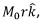where r is the distance from the cylindrical axis. Find the magnetic field both inside and outside the cylinder due to magnetization

3. Repeat Problem 2 by calculating the H field and adding the magnetization contribution.

4. A magnetized material is in the shape of a cube of side a as shown . The magnetization is directed along the z direction and varies linearly from its value zero on its surface on the y-

z plane to a value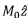on the plane x= α Calculate the bound currents both on the surface and inside the material.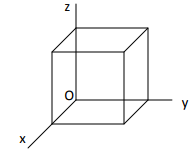Solutions to Self Assessment Questions

1. The bound volume current is zero since the magnetization has constant magnitude. The surface current density is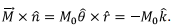The magnetic field inside is zero as there is no volume current inside. The current outside is given by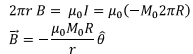2. Bound current densities are as follows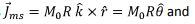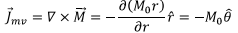By symmetry the fields at The total current enclosed within a length L of the cylinder is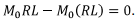Thus the magnetic field outside is zero.

Since the current is in azimuthal direction, in order to calculate the magnetic field, we need to take an Amperian loop which is in the form of a rectangle of height L whose one side is at a distance r from the axis and the other parallel side is outside. Since the field outside is zero, we have,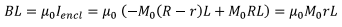which gives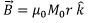3. Since there are no free currents, H=0. Thus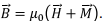Since the magnetization is zero outside, the field is zero. Inside, the field is equal to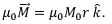4. Since the magnetization varies linearly with x, the magnetization is given by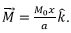The bound current density in the volume is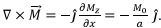The surface bound currents are as follows. On the face x=0, there is no bound current because M=0 on that face. On the face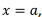the normal direction is î so that the bound current density is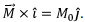On the top face, the normal direction is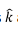and the magnetization is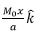so that the bound current density is zero. We have a contribution from the y=α face for which the normal is directed along J and the bound current density is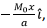the current on the opposite face is oppositely directed.

The document Magnetized Material Notes | Study Electromagnetic Fields Theory - Electronics and Communication Engineering (ECE) is a part of the Electronics and Communication Engineering (ECE) Course Electromagnetic Fields Theory.
All you need of Electronics and Communication Engineering (ECE) at this link: Electronics and Communication Engineering (ECE)Use Code STAYHOME200 and get INR 200 additional OFF

## Electromagnetic Fields Theory

11 videos|46 docs|75 tests

Track your progress, build streaks, highlight & save important lessons and more!

,

,

,

,

,

,

,

,

,

,

,

,

,

,

,

,

,

,

,

,

,

;